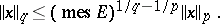# Imbedding of function spaces

(diff) ← Older revision | Latest revision (diff) | Newer revision → (diff)

A set-theoretic inclusion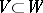of a linear normed spaceinto a linear normed space, for which the following inequality is valid for any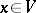: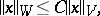whereis a constant which does not depend on. Here,is the norm (semi-norm) of the elementin, whileis the norm (semi-norm) of the elementin.

The identity operator frominto, which assigns to an elementthe same element seen as an element of, is said to be the imbedding operator ofinto. The imbedding operator is always bounded. If the imbedding operator is a completely-continuous operator, the imbedding of function spaces is said to be compact. Facts on imbedding of function spaces are established by so-called imbedding theorems.

Example. Letbe a Lebesgue-measurable set in the-dimensional Euclidean space with finite measureand let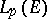,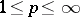, be the Lebesgue space of measurable functions which are-th power summable overwith norm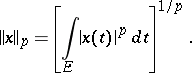Then, if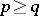, one has the imbedding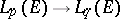, and